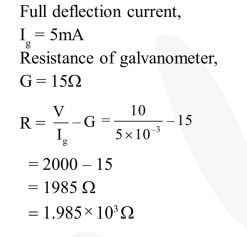# When a current of 5 mA is passed through a galvanometer having a coil of`
Question:

When a current of $5 \mathrm{~mA}$ is passed through a galvanometer having a coil of resistance $15 \Omega$, it shows full scale deflection. The value of the resistance to be put in series with the galvanometer to convert it into to voltmeter of range $0-10 \mathrm{~V}$ is :-

1.  $2.535 \times 10^{3} \Omega$

2. $4.005 \times 10^{3} \Omega$

3. $1.985 \times 10^{3} \Omega$

4.  $2.045 \times 10^{3} \Omega$

Correct Option: , 3

Solution: